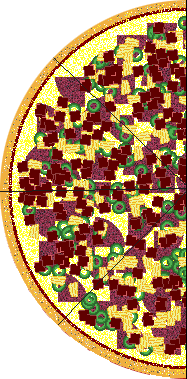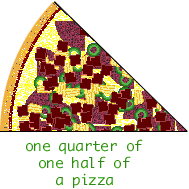Hello! I was looking at your Fraction questions and since i am pursuing to be an elementry school teacher i feel i don't understand one of your responses. How would you explain this to an elem, midd, and high school student. Even me myself-in college really does not understand. The question was: When you multiply two positive fractions less than 1, how does the answer compare to the size of the fraction? Why?

Thank you

Katie

Hi Katie,

A positive fraction less than one is used to represent part of a whole. One fifth of a dollar is part of a dollar and two thirds of a book is part of the book. A second fractional part of that part is smaller yet.

You might try to explain this with something concrete. Suppose I have one half of a pizza and three friends come over. If we split the one half of a pizza then we each get one quarter of one half of a pizza. That is we each get  1/4 x 1/2 = 1/8 of a pizza.I hope this helps,
Penny
Go to Math Central